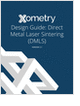Design HomeStandard Beams Steel W Type I-Beam Steel S Type I-Beam Steel Channels Steel Angles Aluminum I-Beams Aluminum ChannelsCommon Beams Square I-Beam Tapered I-Beam Uneven I-Beam General Shape Square Channel Tapered Channel Square L Beam Rectangular L Beam Square T Beam Semi-tapered T Beam Tapered T Beam Rectangular CrossApplications Beam Bending Bibliography
3D Scanners

A white paper to assist in the evaluation of 3D scanning hardware solutions.

Metal 3D Printing Design Guide

Direct Metal Laser Sintering (DMLS) 3D printing for parts with reduced cost and little waste.

Injection Molding Design Guide

Guide for high quality and cost-effective plastic injection molding.

Learn the best principles to negotiate the salary you deserve!

more free magazinesMoment of InertiaMoment Of Inertia Second Moment of Area, Area Moment of Inertia

The Area Moment Of Inertia of a beams cross-sectional area measures the beams ability to resist bending. The larger the Moment of Inertia the less the beam will bend.
The moment of inertia is a geometrical property of a beam and depends on a reference axis. The smallest Moment of Inertia about any axis passes throught the centroid.
The following are the mathematical equations to calculate the Moment of Inertia:
Ixequ. (1)
Iyequ. (2)
y is the distance from the x axis to an infinetsimal area dA.
x is the distance from the y axis to an infinetsimal area dA.

Example

 Polar Moment Of Inertia Moment of Inertia about the z axis

The Polar Area Moment Of Inertia of a beams cross-sectional area measures the beams ability to resist torsion. The larger the Polar Moment of Inertia the less the beam will twist.
The following are the mathematical equations to calculate the Polar Moment of Inertia:
Jzequ. (3)
x is the distance from the y axis to an infinetsimal area dA.
y is the distance from the x axis to an infinetsimal area dA.

 PERPENDICULAR AXIS THEOREM: The moment of inertia of a plane area about an axis normal to the plane is equal to the sum of the moments of inertia about any two mutually perpendicular axes lying in the plane and passing through the given axis.

Using the PERPENDICULAR AXIS THEOREM yeilds the following equations for the Polar Moment of Inertia:
Jz = Ix+Iy equ. (4)

 Example | Radius of Gyration | Parallel Axis Theorem
Glossary3D Scanners

A white paper to assist in the evaluation of 3D scanning hardware solutions.Metal 3D Printing Design Guide

Direct Metal Laser Sintering (DMLS) 3D printing for parts with reduced cost and little waste.Injection Molding Design Guide

Guide for high quality and cost-effective plastic injection molding.